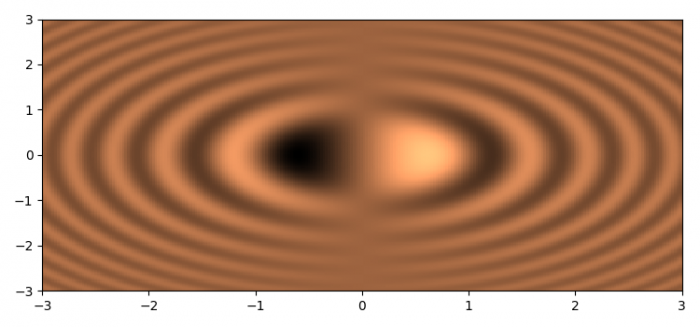# How to visualize scalar 2D data with Matplotlib?

To visualize scalar 2D data with matplotlib, we can take the following steps −

• Set the figure size and adjust the padding between and around the subplots.
• Initialize a variable, N, for data samples.
• Create x and y data points using numpy.
• Get coordinate matrices from coordinate vectors.
• Get z data points using numpy.
• Create a pseudocolor plot with a non-regular rectangular grid.
• To display the figure, use show() method.

## Example

import numpy as np
from matplotlib import pyplot as plt

plt.rcParams["figure.figsize"] = [7.50, 3.50]
plt.rcParams["figure.autolayout"] = True

n = 256

x = np.linspace(-3., 3., n)
y = np.linspace(-3., 3., n)

X, Y = np.meshgrid(x, y)
Z = X * np.sinc(X ** 2 + Y ** 2)

plt.show()